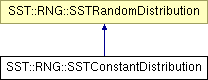# SST::RNG::SSTConstantDistribution Class Reference

Implements a distribution which always returns a constant value (provided by the user). More...

`#include "sst/core/rng/constant.h"`

Inheritance diagram for SST::RNG::SSTConstantDistribution:## Public Member Functions

SSTConstantDistribution (double v)
Creates a constant distribution which returns a constant value.
~SSTConstantDistribution ()
Destroys the constant distribution.
double getNextDouble ()
Gets the next double for the distribution, in this case it will return the constant value specified by the user.
double getMean ()
Gets the constant value for the distribution.

## Protected Attributes

double mean
Describes the constant value to return from the distribution.

## Detailed Description

Implements a distribution which always returns a constant value (provided by the user).

This can be used in situations where the user may not want to apply a distribution.

## Constructor & Destructor Documentation

 SST::RNG::SSTConstantDistribution::SSTConstantDistribution ( double v ) ` [inline]`

Creates a constant distribution which returns a constant value.

Parameters:
 v Is the constant value the user wants returned by the distribution

References mean.

## Member Function Documentation

 double SST::RNG::SSTConstantDistribution::getMean ( ) ` [inline]`

Gets the constant value for the distribution.

Returns:
Constant value specified by the user when creating the class

References mean.

 double SST::RNG::SSTConstantDistribution::getNextDouble ( ) ` [inline, virtual]`

Gets the next double for the distribution, in this case it will return the constant value specified by the user.

Returns:
Constant value specified by the user when creating the class

Implements SST::RNG::SSTRandomDistribution.

References mean.

The documentation for this class was generated from the following file:

Generated on 14 Sep 2015 for SST by1.6.1